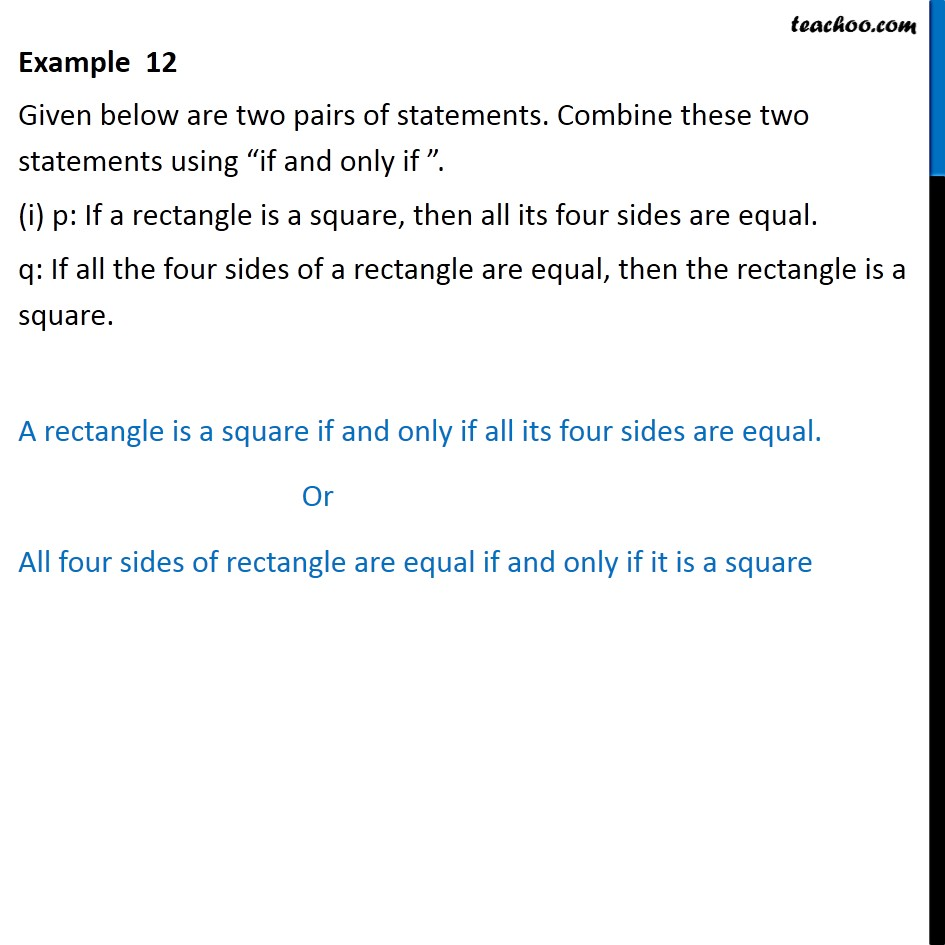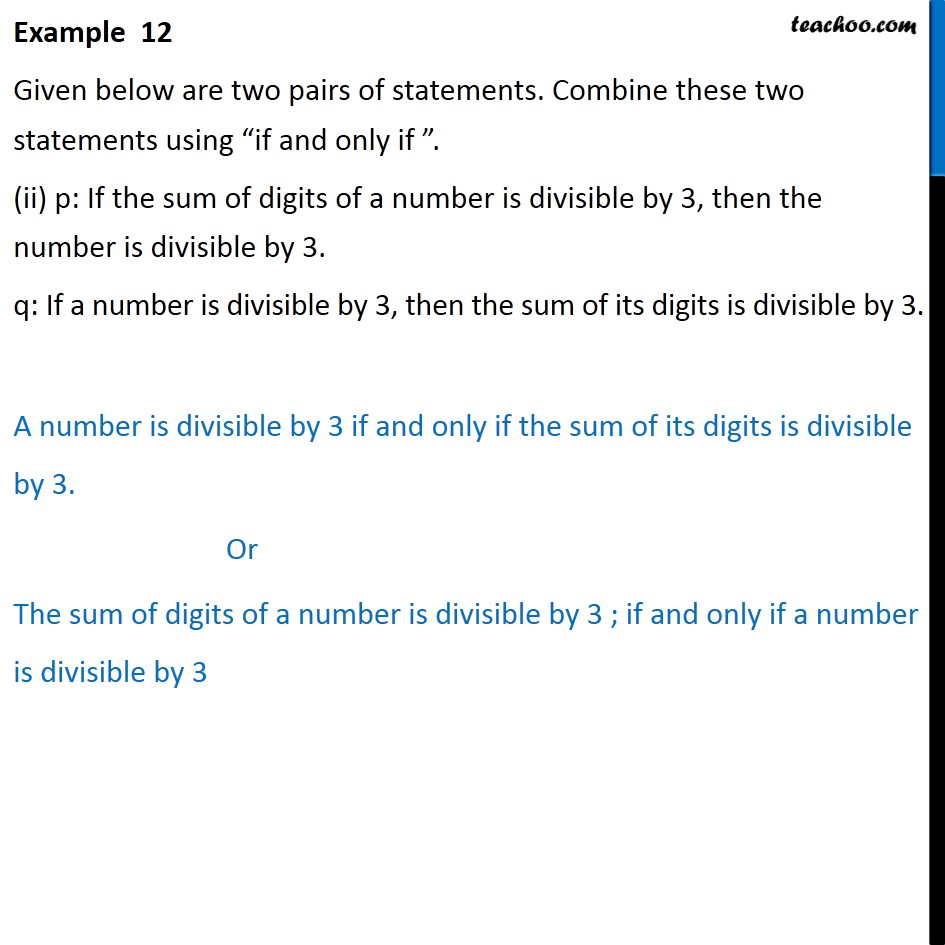Subscribe to our Youtube Channel - https://you.tube/teachoo

1. Chapter 14 Class 11 Mathematical Reasoning
2. Serial order wise
3. Examples

Transcript

Example 12 Given below are two pairs of statements. Combine these two statements using if and only if . (i) p: If a rectangle is a square, then all its four sides are equal. q: If all the four sides of a rectangle are equal, then the rectangle is a square. A rectangle is a square if and only if all its four sides are equal. Or All four sides of rectangle are equal if and only if it is a square Example 12 Given below are two pairs of statements. Combine these two statements using if and only if . (ii) p: If the sum of digits of a number is divisible by 3, then the number is divisible by 3. q: If a number is divisible by 3, then the sum of its digits is divisible by 3. A number is divisible by 3 if and only if the sum of its digits is divisible by 3. Or The sum of digits of a number is divisible by 3 ; if and only if a number is divisible by 3

Examples